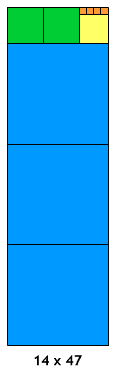# Approximations, Euclid's Algorithm & Continued Fractions

##### Age 16 to 18

Published June 2001,February 2011.

In this article we see how Euclid's algorithm can be used to produce continued fractions and how these continued fractions can be truncated after a very few steps to give close rational approximations to irrational numbers.

In earlier articles we have seen a geometric interpretation of Euclid's algorithm in which we divide a rectangle up into squares plus a smaller rectangle and then divide the smaller rectangle into squares plus an even smaller rectangle and so on until we finally get to a rectangle that is completely made up of squares.

See the article Euclid's Algorithm I.

We have also discussed continued fractions. If you have not read the earlier articles, it might be a good idea to look at them before you read this one.

These articles are Continued Fractions I and Continued Fractions II .

We start by evaluating the following continued fraction: $$3+ {1 \over \displaystyle 2\;+\; { 1 \over \displaystyle 1\;+\; { 1\over \displaystyle 4}}}.$$ The value of this is $$3+{1\over\displaystyle 2+ { 4 \over \displaystyle 5}} = 3+ {5\over\displaystyle 14}= {47\over\displaystyle 14}.$$ Now draw (on graph paper) a rectangle of base 14 units and height 47 units; we shall call this a $14\times 47$ rectangle.It is easy to see that this rectangle can be divided into THREE $14\times 14$ squares and one $5\times 14$ rectangle. Draw these squares inside the $14\times 47$ rectangle, starting from the bottom. Now take the $5\times 14$ rectangle and divide this into TWO $5\times 5$ squares, and one $4\times 5$ rectangle. Again, you should draw these squares in your diagram. Finally, the $4\times 5$ rectangle can be divided into ONE $4\times 4$ square and one $1\times 4$ rectangle which can itself be divided into FOUR $1\times 1$ squares. Notice that the number of squares found at each stage (written in capital letters) are exactly the numbers appearing in the continued fraction (which has $1$ at the top of each fraction).   Let us try another example: $$2+ {1\over\displaystyle 1+ { 1 \over \displaystyle 2+ { 1\over \displaystyle 3}}}= 2+{1\over\displaystyle 1+ { 3 \over \displaystyle 7 }} = 2+ {7\over\displaystyle 10} = { 27 \over \displaystyle 10}.$$ If we follow the instructions given above, we start with a $10\times 27$ rectangle which we divide into TWO $10\times 10$ squares and a $7\times 10$ rectangle. This last rectangle is divided into ONE $7\times 7$ square and a $3\times 7$ rectangle. The $3\times 7$ rectangle divides into TWO $3\times 3$squares and a $1\times 3$ rectangle which then divides into THREE $1\times 1$ squares.

We can now try and reverse this process, and this will give us a way of finding the continued fraction for a given ratio. For example, starting with the number $43/30$ we construct a $30\times 43$ rectangle (again, you should use graph paper here). Now divide this into a number of $30\times 30$ squares (how many?) and a rectangle (of what size?) If you continue in this way you should arrive at the continued fraction expansion for $43/30$, and you can then check your answer by working out the continued fraction as we have done above. Of course, if your work is correct, the value of the continued fraction you get will be $43/30$.

Here are two more examples to try. First start with the fraction $43/20$, draw the squares and rectangles as above; then find the continued fraction for $43/20$ , and check that it really does have the value $43/20$. Now do the same with $43/10$.

Euclid's algorithm is best known for finding the highest common factor of two whole numbers, which is then used in solving Diophantine equations such as $ax + by = c$ where $a, b$ and $c$ are integers. Note that when the lengths of the edges of the original rectangle are whole numbers, because the rectangles produced by Euclid's algorithm get smaller and smaller and still have edges whose lengths are whole numbers, finally the process must terminate with a rectangle with an edge of 1 unit.
Suppose for example you want to find a rational approximation to $\pi$. We know that ${22\over 7}$ is used as an approximation but where did that come from, and can we find a better rational approximation? To 7 decimal places $\pi \approx 3.1415927$ which gives ${31415927\over 10000000}$ as a rational approximation but we can do much better than that.
Taking the reciprocal of 0.1415927 we have $$\pi \approx 3+ {1\over\displaystyle 7.0625133}$$ which gives the rational approximation $\pi \approx 3 + {1\over 7} = {22\over 7}$ giving an approximation accurate to 3 significant figures.
For a better approximation, taking the reciprocal of 0.0625133 we have $$\pi \approx 3+ {1\over\displaystyle 7 + {1\over \displaystyle 15.9966}}.$$ Repeating this algorithm we have $$\pi \approx 3+ {1\over \displaystyle 7 + {1\over \displaystyle 15 + {1\over \displaystyle 1 + {1\over \displaystyle 293 + {1\over \displaystyle 10.320556}}}}}$$ and so on ... This gives the approximation (which is accurate to 7 significant figures): $$\pi \approx 3+ {1\over \displaystyle 7 + {1\over \displaystyle 15 + 1}} = {3 + {16\over 113}} = {355\over 113}.$$